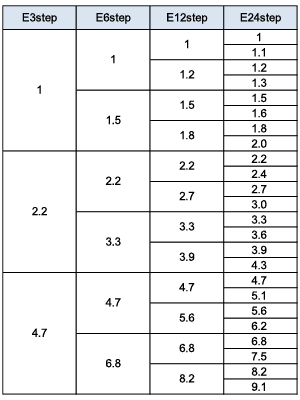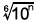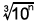# Ceramic Capacitors FAQ

Characteristics

How is the capacitance value of a capacitor obtained?

The capacitance value of a capacitor is determined by the number of value steps (E steps) as follows.
E steps include E3 step, E6 step, E12 step, E24 step and so on, and are determined as follows according to the JIS Standard.
(From JISC5063)-----------------------------------------------------------------------------------------
The preferred number series for E6 consists of the numbers obtained by rounding the logical number of, and comprises alternating numbers in the E12 number series.
The preferred number series for E3 consists of the numbers obtained by rounding the logical number of, and comprises alternating numbers in the E6 number series.
-----------------------------------------------------------------------------------------
For example, in the case of E3 step, there are three steps until the digit increases by one in the manner of 1, 2.2, 4.7, 10, 22, 47, 100 and so on.

The standard steps for Murata multilayer ceramic capacitors are as follows.
10 pF or less: 0.1-pF increments
10 pF to 1 uF: E6 step
1 uF or more: E3 step
* E12 step and E24 step are also used according to the temperature characteristics and the series.